Courses

# Subjective Questions of Electromagnetic Induction and Alternating Current (Part -1) JEE Notes | EduRev

## Class 12 Physics 35 Years JEE Mains &Advance Past year Paper

Created by: Abhishek Kapoor

## JEE : Subjective Questions of Electromagnetic Induction and Alternating Current (Part -1) JEE Notes | EduRev

The document Subjective Questions of Electromagnetic Induction and Alternating Current (Part -1) JEE Notes | EduRev is a part of the JEE Course Class 12 Physics 35 Years JEE Mains &Advance Past year Paper.
All you need of JEE at this link: JEE

Q.1. A current from A to B is increasing in magnitude. What is the direction of induced current, if any, in the loop as shown in the figure?        (1979)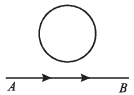Ans. Clockwise

Solution. The magnetic lines of force due to current flowing in wire AB is shown.

NOTE : As the current increases, the number of magnetic lines of force passing through the loop increases in the outward direction. To oppose this change, the current will flow in the clockwise direction.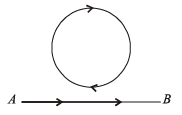Q.2. The two rails of a railway track, insulated from each other and the ground, are connected to a milli voltmeter. What is the reading of the milli voltmeter when a train travels at a speed of 180 km/hour along the track, given that the vertical component of earth’s magnetic field is 0.2 × 10–4 weber/m2 & the rails are separated by 1 meter?     (1981- 4 Marks)

Ans. 1 mV

Solution. KEY CONCEPT :  This is based on motional emf.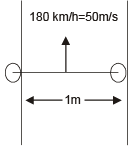e = vBℓ = 50 × 0.2 × 10–4 × 1  = 10–3 volt  = 1 milli volt

Q.3. Three identical closed coils A, B and C are placed with their planes parallel to one another. Coils A and C carry equal currents as shown in Fig. Coils B and C are fixed in position and coil  A  is moved towards B with uniform motion. Is there any induced current in B ? If no, give reasons. If yes mark the direction of the induced current in the diagram.     (1982 - 2 Marks)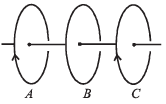Ans. Yes, opposite direction of A

Solution.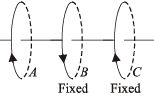NOTE : When the coil A moves towards B, the number of magnetic lines of force passing through B changes.

Therefore, an induced emf and hence induced current is produced in B.

The direction of current in B will be such as to oppose the field change in B and therefore, will be in the opposite direction of A.

Q.4. A square metal wire loop of side 10 cms and resistance 1ohm is moved with a constant velocity v0 in a uniform magnetic field of induction B = 2 webers/ m2 as shown in the figure. The  magnetic field lines  are perpendicular to the plane of the  loop (directed into the paper).  The loop is connected to a network of  resistors each of value 3 ohms.  The resistances of the lead wires OS and PQ are negligible. What should be the  speed of the loop so as to have a steady current of 1 milliampere in the  loop ?

Give the direction of current in the loop.      (1983 - 6 Marks)

Ans. 0.02 m/s, clockwise direction

Solution.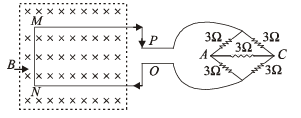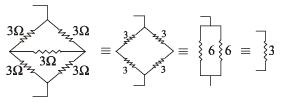NOTE : The network behaves like a balanced wheatstone bridge.

The free electrons in the portion MN of the rod have a velocity v in the right direction. Applying Fleming’s left hand rule, we find that the force on electron will be towards N.

Hence, M will be + ve and N will be negative. Current will flow in clockwise direction.

The induced emf developed is given by

e = vBℓ = v × 2 ×0.1 = 0.2v ...(i)

Now, e = IR

e = 10 – 3 × 4 = 4 ×10–3 amp ...(ii)

From (i) and (ii),

0.2 v = 4 ×10 –3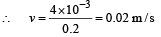Q.5. Space is divided by the line AD into two regions. Region I is field free and the Region II has a uniform magnetic field B directed into the plane of the paper. ACD is a semicircular conducting loop of radius r with centre at O, the plane of the loop being in the plane of the paper. The loop is now made to rotate with a constant angular velocity w about an axis passing through O and the perpendicular to the plane of the paper. The effective resistance of the loop is R.           (1985 - 6 Marks)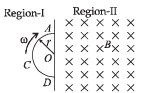(i) Obtain an expression for the magnitude of the induced current in the loop.
(ii) Show the direction of the current when the loop is entering into the Region II.
(iii) Plot a graph between the induced e.m.f and the time of rotation for two periods of rotation.

Ans.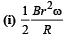(ii) anticlockwise

Solution. (i) Induced emf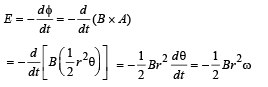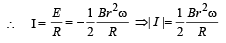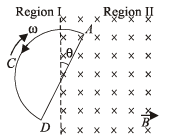(ii) The loop is entering in the magnetic field and hence magnetic lines of force passing through the loop is increasing in the downward direction. Therefore, current will flow in the loop in such a direction which will oppose the change. The current will flow in the anticlockwise direction.
(iii) Graph between induced emf and period of rotation: For first half rotation, (t = T/2), when the loop enters the field, the current is in anticlockwise direction. Magnitude of current remains constant at I = Bωr2 /2R .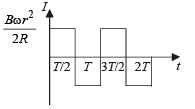For next half rotation, when the loop comes out of the field, current of the same magnitude is set up clockwise.

Anticlockwise current is supposed to be positive. The I-t graph is shown in the figure for two periods of rotation.

Q.6. Two long parallel horizontal rails, a distance d apart and each having a resistance λ per unit length, are joined at one end by a resistance R. A perfectly conducting rod MN of mass m is free to slide along the rails without friction (see figure). There is a uniform magnetic field of induction B normal to the plane of the paper and directed into the paper.

A variable force F is applied to the rod MN such that, as the rod moves, a constant current flows through R.         (1988 - 6 Marks)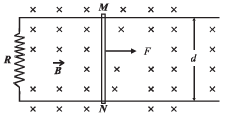(i) Find the velocity of the rod and the applied force F as function of the distance x of the rod from R.
(ii) What fraction of the work done per second by F is converted into heat ?

Ans.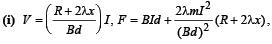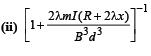Solution. (i) A variable force F is applied to the rod MN such that as the rod moves in the uniform magnetic field a constant current flows through R. Consider the loop MPQN.

Let MN be at a distance x from PQ.

Length of rails in loop = 2x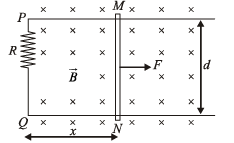∴ Resistance of rails in loop = 2xλ

∴ Total resistance of loop = R + 2λx

Induced emf = Bvd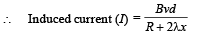So for constant I,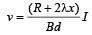..(i)

Furthermore, as due to induced current I the wire will experience a force FM = BId opposite to its motion, the equation of motion of the wire will be

F – FM = ma     i.e.,     F = FM + ma

But as here FM = BId and from equation (i)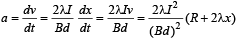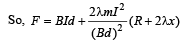(ii) As the work done by force F per sec.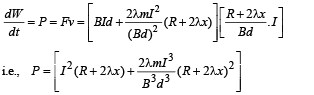and heat produced per second, i.e., joule heat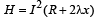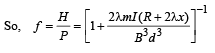Q.7. A circuit containing a two position switch S is shown in fig.    (1991 - 4 + 4  Marks)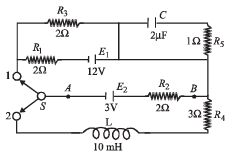(a) The switch S is in position ‘1’. Find the poten tial difference VA – VB and the rate of production of joule heat in R1.
(b) If now  the switch S is put in position 2 at t = 0 find
(i)   steady current in R4 and
(ii)  the time when current in R4 is  half the steady value.

Also calculate the energy stored in the inductor L at that time

Ans. (a)  –5V, 24.5 W
(b) (i) 0.6 amp.

(ii) 1.386 × 10–3 sec., 4.5 × 10–4 J

Solution. (a) (i) In this case S and I are connected.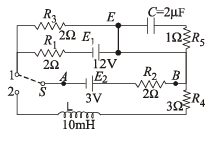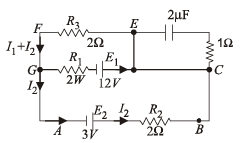Using Kirchhoff’s law in ABCDGA

+ 3 – I2 × 2 – 12 + I1× 2 = 0

2 I1 – 2I2 = 9 ...(i)

Applying Kirchhoff ’s law in DEFGD

– 2I1 + 12 – (I1 + I2) 2 = 0

⇒ 2I1 + I2 = 6 ...(ii)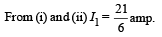∴ From (ii)  I2 = –1 amp.

To find potential difference between A and B

VA + 3 – (– 1) × 2 = VB ⇒ VA – VB = – 5V

The rate of production of heat in R1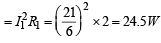(b) (i)  When the switch is put in position 2 then the active circuit will be as shown in the figure.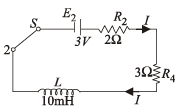When the steady state current is reached then the inductor plays no role in the circuit

E2 = I (R2 + R4)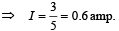(ii) KEY CONCEPT : The growth of current in L–R circuit is given by the expression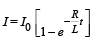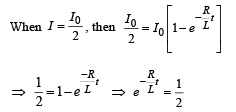Taking log on both sides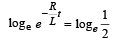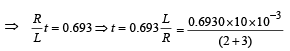when R = R2 + R4

⇒ t = 1.386 × 10 –3 sec.

Thus this much time is required for current to reach half of its steady value.

The energy stored by the inductor at that time is given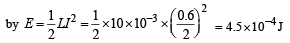Q.8. A rectangular frame ABCD, made of a uniform metal wire, has a straight connection between E and F made of the same wire, as shown in Fig.  AEFD is a square of side 1m, and EB = FC = 0.5m. The entire circuit is placed in steadily increasing, uniform magnetic field directed into the plane of the paper and normal to it.

The rate of change of the magnetic field is 1T/s. The resistance per unit length of the wire is 1Ω / m. Find the magnitudes and directions of the currents in the segments AE, BE and EF.       (1993-5 Marks)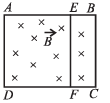Ans.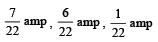Solution. The equivalent circuit is drawn in the adjacent figure.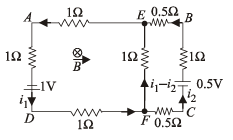NOTE : As the magnetic field increases in the downward direction, an induced emf will be produced in the AEFD as well as in the circuit EBCF such that the  current flowing in the loop creates magnetic lines of force in the upward direction (to the plane of paper).

Thus, the current should flow in the anticlockwise direction in both the loops.

Induced emf in loop AEFD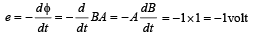Induced emf in loop EBCF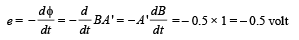Let the current flowing in the branch EADF be i1 and the current flowing in the branch FCBE be i2. Applying junction law at F, we get current in branch FE to be (i1 – i2) Applying Kirchhoff ’s law in loop EADFE

– 1 × i1 – 1× i1 + 1 – 1 × i1 – 1 (i1 – i2) = 0

⇒ 4i1 – i2 = 1       ... (i)

Applying Kirchhoff 's law in loop EBCFE

+ 0.5i1 – 0.5 + 1i2 + 0.5 i2 – 1(i1 – i2) = 0

– i1 + 3i2 = 0.5         ... (ii)

Solving (i) and (ii)

11 i1 = 3.5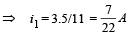Also 11i2 = 3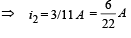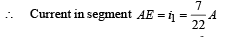Q.9. Two parallel vertical metallic rails AB and CD are separated by 1 m. They are connected at two ends by resistances R1 and R2 as shown in Figure.  A horizontal metallic bar  L of mass 0.2 kg slides without friction vertically down the rails under the action of gravity. There is a uniform horizontal magnetic field of 0.6 Tesla perpendicular to the plane of the rails. It is observed that when the terminal velocity is attained, the powers dissipated in R1 and R2 are 0.76 Watt and 1.2 watt respectively. Find the terminal velocity of the bar L and the values of R1 and R2.     (1994 - 6 Marks)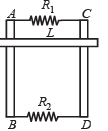Ans. 1 m/s, 0.47 Ω, 0.3 Ω

Solution. KEY CONCEPT : We can understand the direction of flow of induced currents by imagining a fictitious battery to be attached between E and F. The direction of induced current can be found with the help of Lenz’s law.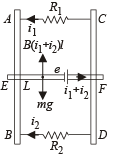NOTE : P. d. across parallel combinations remains the same

Also, P1 = ei1 = 0.76 W and P2 = ei2 = 1.2 W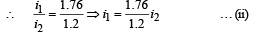The horizontal metallic bar L moves with a terminal velocity.

This means that the net force on the bar is zero.

∴ B (i1 + i2) = mg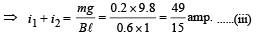From (ii) and (iii)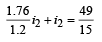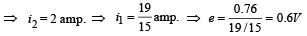The induced emf across L due to the movement of bar L in a magnetic field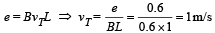Also from (i),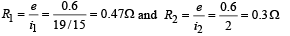Q.10. A metal rod OA of mass ‘m’ and length ‘r’ is kept rotating with a constant angular speed ω in a vertical plane about a horizontal axis at the end O. The free end A is arranged to slide without friction along a fixed conducting circular ring in the same plane as that of rotation. A uniform and constant magnetic induction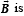applied perpendicular and into the plane of rotation as shown in the figure below. An inductor L and an external resistance R are connected through a switch S between the point O and a point C on the ring to form an electrical circuit. Neglect the resistance of the ring and the rod. Initially, the switch is open.                          (1995 - 10 Marks)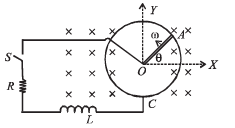(a) What is the induced emf across the terminals of the switch?

(b) The switch S is closed at time t = 0.

(i) Obtain an expression for the current as a function of time.

(ii) In the steady state, obtain  the time dependence of the torque required to maintain the constant angular speed, given that the rod OA was along the positive X-axis at t = 0.

Ans.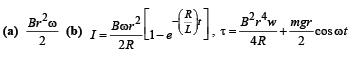Solution. (i) (a) Let us consider a small length of metal rod dx at a distance x from the origin. Small amount of emf (de) induced in this small length (due to metallic rod cutting magnetic lines of force) is

de = B (dx) v         … (i)

where v is the velocity of small length

dxv = xω        … (ii)

∴ The total emf acoss the whole metallic rod OA is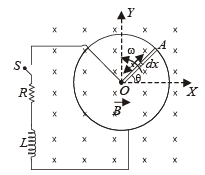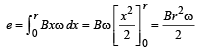(b) The above diagram can be reconstructed as the adjacent figure. e is a constant. O will accumulate positive charge and A negative. When the switch S is closed, transient current at any time t, when current I is flowing in the circuit,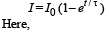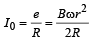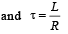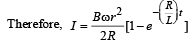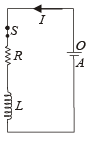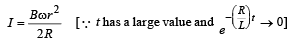NOTE : When current flows in the circuit in steady state, there is a power loss through the resistor.

Also since the rod is rotating in a vertical plane, work needs to be done to keep it at constant angular speed.

Power loss due to current I will be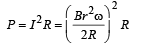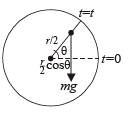If torque required for this power is τ1 then

P = τ1ω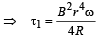Torque required to move the rod in circular motion against gravitational field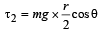The total torque

τ = τ1 + τ2 (Clockwise)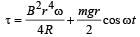The required torque will be of same magnitude and in anticlockwise direction. The second term will change signs as the value of cos θ can be positive as well as negative.

Offer running on EduRev: Apply code STAYHOME200 to get INR 200 off on our premium plan EduRev Infinity!

42 docs|19 tests

,

,

,

,

,

,

,

,

,

,

,

,

,

,

,

,

,

,

,

,

,

;